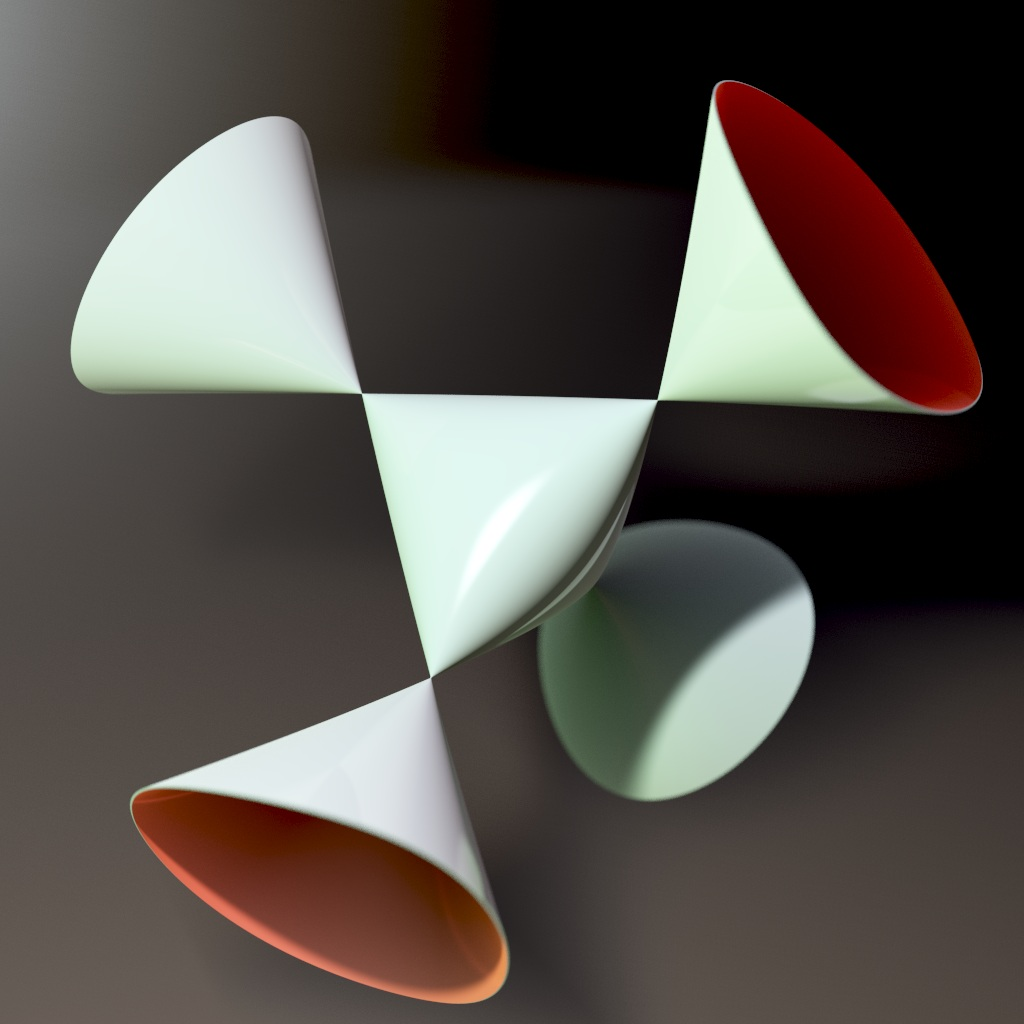# Cayley’s Nodal Cubic Surface

A cubic surface is one defined by a polynomial equation of degree 3. A nodal surface is one whose only singularities are ordinary double points: that is, points where it looks like the origin of the cone in 3-dimensional space defined by

$$x^2 + y^2 = z^2 .$$

Cayley’s nodal cubic surface, drawn above by Abdelaziz Nait Merzouk, is the cubic surface with the largest possible number of ordinary double points, namely 4. In fact, every cubic with 4 ordinary double points is isomorphic to this one.Cayley’s Nodal Cubic Surface (Cut Version) – Abdelaziz Nait Merzouk

Cayley’s nodal cubic surface is described by this equation

$$wxy + wxz + wyz + xyz = 0$$

This equation determines a subset $S \subset \mathbb{C}^4$ with complex dimension 2. Note that if $(w, x, y, z \in \mathbb{C}^4$ is a solution, so is any multiple $(cw, cx, cy, cz)$. We may thus projectivize $S$, treating any solution as ‘the same’ as any multiple of that solution. The result is an algebraic variety $X$ in the complex projective space $\mathbb{C}\mathrm{P}^3$. This variety has complex dimension 2, so it is called a complex surface. To obtain an ordinary real 2-dimensional surface we may take its intersection with a copy of $\mathbb{R}\mathrm{P}^3$ in $\mathbb{C}\mathrm{P}^3$.

Sitting inside $\mathbb{R}\mathrm{P}^3$ we in turn have many copies of ordinary 3-dimensional space, $\mathbb{R}^3$. The pictures above show the portion of Cayley’s nodal cubic surface living in one of these copies.

The simple double points in Cayley’s nodal cubic occur where three of the coordinates $w,x,y,z$ are zero. The hyperplane $w + x + y + z = 1$ determines a copy of $\mathbb{C}^3$ inside $\mathbb{C}\mathrm{P}^3$, and taking all four coordinates to be real gives a copy of $\mathbb{R}^3$ in which these double points lie at the vertices of a regular tetrahedron. Indeed, the symmetry group of Cayley’s nodal cubic is $\mathrm{S}_4$, the symmetry group of a tetrahedron.

Puzzle 1. There are 9 lines on Cayley’s nodal cubic surface. 6 of these lines contain the edges of the tetrahedron described above. What are the other 3 lines?

Some interesting properties of Cayley’s nodal cubic surface are discussed here:

• Bruce Hunt, Nice modular varieties, Experimental Mathematics 9 (2000), 613–622.

In particular, he explains how it is a compactification of a quotient of a ball, and a moduli space for certain abelian fourfolds.

Puzzle 2. Show that after a change of variables, Cayley’s nodal cubic surface can also be described by the equation

$$w^3 + x^3 + y^3 + z^3 = (w + x + y + z)^3$$

Visual Insight is a place to share striking images that help explain advanced topics in mathematics. I’m always looking for truly beautiful images, so if you know about one, please drop a comment here and let me know!

## 3 thoughts on “Cayley’s Nodal Cubic Surface”

1.Matthew Arcus says:

You might like my WebGL animation of Cayley’s cubic (as well as the Clebsch cubic – complete with 27 lines – and a surface that morphs from one to the other):

https://matthewarcus.github.io/polyjs/#cubics

•John Baez says:

Those are really cool! I like that style of animation even though the swarms of tiny spheres are a bit disturbing.

2.Michael Janas says:

Puzzle 2 is not actually correct as written: It should be 4(x^3+y^3+z^3+w^3)=(x+y+z+w)^3. Without that factor of 4, the resulting cubic has no nodes and so cannot be Cayley’s nodal cubic.

My own preferred version FWIW is the affine form 1+2XYZ = X^2+Y^2+Z^2, arising as the determinant of a symmetric matrix with ones on the diagonal and off-diagonal elements X,Y,Z. As a consequence, one may readily show that the middle portion of the curve and its interior parametrize the set of 3-by-3 correlation matrices (PSD with ones on the diagonal) and so can be called the 3-elliptope.